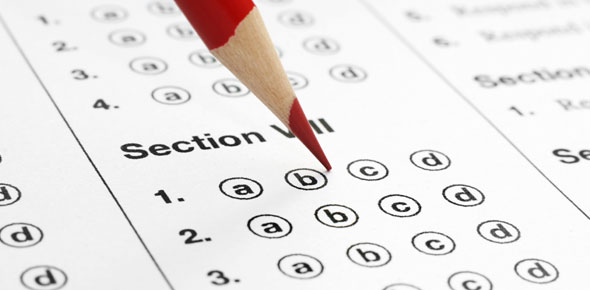# Quantitative Apptitude - Average Online Test 7

10 Questions | Attempts: 1706
ShareSettings• 1.
Find the average of all prime numbers between 30 and 50?
• A.

39

• B.

39.8

• C.

40.8

• D.

37.8

• 2.
The average of first five multiples of 3 is?
• A.

6

• B.

9

• C.

15

• D.

3

• 3.
The average weight of a class of 30 students is 40 kgs if the teacher weight is included then average increases by 2 kgs then find the weight of the teacher?
• A.

96

• B.

100

• C.

102

• D.

106

• 4.
The captain of a cricket team of 11 members is 26 years old and the wicket keeper is 3 years older. If the ages of these two are excluded, the average age of the remaining players is one year less than the average age of the whole team. What is the average age of the team?
• A.

23

• B.

25

• C.

27

• D.

22

• 5.
Find the average of first 40 natural numbers?
• A.

20

• B.

21

• C.

19

• D.

20.5

• 6.
Find the average of first 20 multiples of 7?
• A.

72

• B.

72.5

• C.

70

• D.

73.5

• 7.
A student was asked to find the arithmetic mean of the numbers 3, 11, 7, 9, 15, 13, 8, 19, 17, 21, 14 and x. He found the mean to be 12. What should be the number in the place of x ?
• A.

5

• B.

7

• C.

17

• D.

9

• 8.
The average age of Mr and Mrs Sharma 4 years ago is 28 years . If the present average age of Mr and Mrs Sharma and their son is 22 years. What is the age of their son?
• A.

1yr

• B.

2yr

• C.

3yr

• D.

4yr

• 9.
The average age of husband, wife and their child 3 years ago was 27 years and that of wife and the child 5 years ago was 20 years. The present age of the husband is?
• A.

35

• B.

40

• C.

45

• D.

50

• 10.
The average of four consecutive even numbers is 27. find the largest of these numbers?
• A.

27

• B.

29

• C.

30

• D.

31

## Related TopicsBack to top
×

Wait!
Here's an interesting quiz for you.